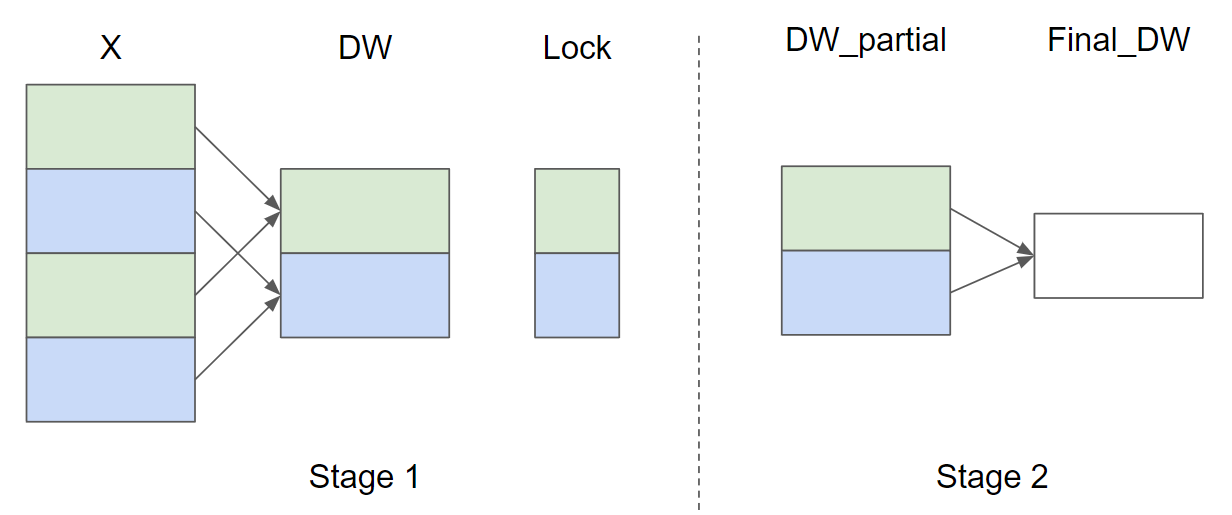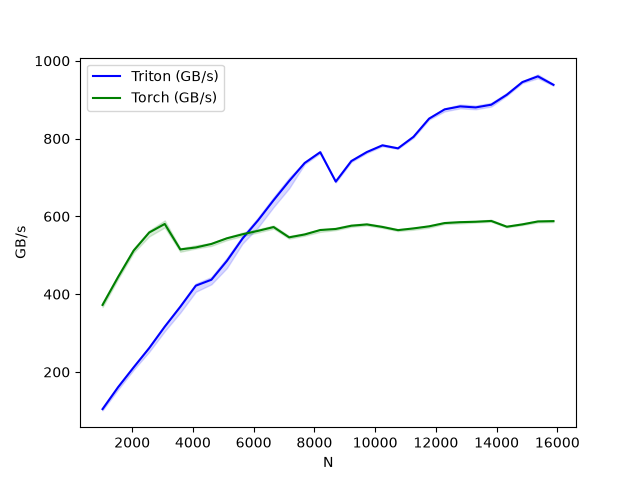# Layer Normalization¶

In this tutorial, you will write a high-performance layer normalization kernel that runs faster than the PyTorch implementation.

In doing so, you will learn about:

• Implementing backward pass in Triton.

• Implementing parallel reduction in Triton.

## Motivations¶

The LayerNorm operator was first introduced in [BA2016] as a way to improve the performance of sequential models (e.g., Transformers) or neural networks with small batch size. It takes a vector $$x$$ as input and produces a vector $$y$$ of the same shape as output. The normalization is performed by subtracting the mean and dividing by the standard deviation of $$x$$. After the normalization, a learnable linear transformation with weights $$w$$ and biases $$b$$ is applied. The forward pass can be expressed as follows:

$y = \frac{ x - \text{E}[x] }{ \sqrt{\text{Var}(x) + \epsilon} } * w + b$

where $$\epsilon$$ is a small constant added to the denominator for numerical stability. Let’s first take a look at the forward pass implementation.

import torch

import triton
import triton.language as tl

try:
# This is https://github.com/NVIDIA/apex, NOT the apex on PyPi, so it
# should not be added to extras_require in setup.py.
import apex
HAS_APEX = True
except ModuleNotFoundError:
HAS_APEX = False

@triton.jit
def _layer_norm_fwd_fused(
X,  # pointer to the input
Y,  # pointer to the output
W,  # pointer to the weights
B,  # pointer to the biases
Mean,  # pointer to the mean
Rstd,  # pointer to the 1/std
stride,  # how much to increase the pointer when moving by 1 row
N,  # number of columns in X
eps,  # epsilon to avoid division by zero
BLOCK_SIZE: tl.constexpr,
):
# Map the program id to the row of X and Y it should compute.
row = tl.program_id(0)
Y += row * stride
X += row * stride
# Compute mean
mean = 0
_mean = tl.zeros([BLOCK_SIZE], dtype=tl.float32)
for off in range(0, N, BLOCK_SIZE):
cols = off + tl.arange(0, BLOCK_SIZE)
_mean += a
mean = tl.sum(_mean, axis=0) / N
# Compute variance
_var = tl.zeros([BLOCK_SIZE], dtype=tl.float32)
for off in range(0, N, BLOCK_SIZE):
cols = off + tl.arange(0, BLOCK_SIZE)
x = tl.where(cols < N, x - mean, 0.)
_var += x * x
var = tl.sum(_var, axis=0) / N
rstd = 1 / tl.sqrt(var + eps)
# Write mean / rstd
tl.store(Mean + row, mean)
tl.store(Rstd + row, rstd)
# Normalize and apply linear transformation
for off in range(0, N, BLOCK_SIZE):
cols = off + tl.arange(0, BLOCK_SIZE)
x_hat = (x - mean) * rstd
y = x_hat * w + b
# Write output


## Backward pass¶

The backward pass for the layer normalization operator is a bit more involved than the forward pass. Let $$\hat{x}$$ be the normalized inputs $$\frac{ x - \text{E}[x] }{ \sqrt{\text{Var}(x) + \epsilon} }$$ before the linear transformation, the Vector-Jacobian Products (VJP) $$\nabla_{x}$$ of $$x$$ are given by:

$\nabla_{x} = \frac{1}{\sigma}\Big( \nabla_{y} \odot w - \underbrace{ \big( \frac{1}{N} \hat{x} \cdot (\nabla_{y} \odot w) \big) }_{c_1} \odot \hat{x} - \underbrace{ \frac{1}{N} \nabla_{y} \cdot w }_{c_2} \Big)$

where $$\odot$$ denotes the element-wise multiplication, $$\cdot$$ denotes the dot product, and $$\sigma$$ is the standard deviation. $$c_1$$ and $$c_2$$ are intermediate constants that improve the readability of the following implementation.

For the weights $$w$$ and biases $$b$$, the VJPs $$\nabla_{w}$$ and $$\nabla_{b}$$ are more straightforward:

$\nabla_{w} = \nabla_{y} \odot \hat{x} \quad \text{and} \quad \nabla_{b} = \nabla_{y}$

Since the same weights $$w$$ and biases $$b$$ are used for all rows in the same batch, their gradients need to sum up. To perform this step efficiently, we use a parallel reduction strategy: each kernel instance accumulates partial $$\nabla_{w}$$ and $$\nabla_{b}$$ across certain rows into one of $$\text{GROUP_SIZE_M}$$ independent buffers. These buffers stay in the L2 cache and then are further reduced by another function to compute the actual $$\nabla_{w}$$ and $$\nabla_{b}$$.

Let the number of input rows $$M = 4$$ and $$\text{GROUP_SIZE_M} = 2$$, here’s a diagram of the parallel reduction strategy for $$\nabla_{w}$$ ($$\nabla_{b}$$ is omitted for brevity):In Stage 1, the rows of X that have the same color share the same buffer and thus a lock is used to ensure that only one kernel instance writes to the buffer at a time. In Stage 2, the buffers are further reduced to compute the final $$\nabla_{w}$$ and $$\nabla_{b}$$. In the following implementation, Stage 1 is implemented by the function _layer_norm_bwd_dx_fused and Stage 2 is implemented by the function _layer_norm_bwd_dwdb.

@triton.jit
def _layer_norm_bwd_dx_fused(DX,  # pointer to the input gradient
DY,  # pointer to the output gradient
DW,  # pointer to the partial sum of weights gradient
DB,  # pointer to the partial sum of biases gradient
X,  # pointer to the input
W,  # pointer to the weights
B,  # pointer to the biases
Mean,  # pointer to the mean
Rstd,  # pointer to the 1/std
Lock,  # pointer to the lock
stride,  # how much to increase the pointer when moving by 1 row
N,  # number of columns in X
eps,  # epsilon to avoid division by zero
GROUP_SIZE_M: tl.constexpr, BLOCK_SIZE_N: tl.constexpr):
# Map the program id to the elements of X, DX, and DY it should compute.
row = tl.program_id(0)
cols = tl.arange(0, BLOCK_SIZE_N)
X += row * stride
DY += row * stride
DX += row * stride
# Offset locks and weights/biases gradient pointer for parallel reduction
lock_id = row % GROUP_SIZE_M
Lock += lock_id
Count = Lock + GROUP_SIZE_M
DW = DW + lock_id * N + cols
DB = DB + lock_id * N + cols
# Compute dx
xhat = (x - mean) * rstd
wdy = w * dy
c1 = tl.sum(xhat * wdy, axis=0) / N
c2 = tl.sum(wdy, axis=0) / N
dx = (wdy - (xhat * c1 + c2)) * rstd
# Write dx
# Accumulate partial sums for dw/db
partial_dw = (dy * xhat).to(w.dtype)
partial_db = (dy).to(w.dtype)
while tl.atomic_cas(Lock, 0, 1) == 1:
pass
# First store doesn't accumulate
if count == 0:
tl.atomic_xchg(Count, 1)
else:
# Release the lock
tl.atomic_xchg(Lock, 0)

@triton.jit
def _layer_norm_bwd_dwdb(DW,  # pointer to the partial sum of weights gradient
DB,  # pointer to the partial sum of biases gradient
FINAL_DW,  # pointer to the weights gradient
FINAL_DB,  # pointer to the biases gradient
M,  # GROUP_SIZE_M
N,  # number of columns
BLOCK_SIZE_M: tl.constexpr, BLOCK_SIZE_N: tl.constexpr):
# Map the program id to the elements of DW and DB it should compute.
pid = tl.program_id(0)
cols = pid * BLOCK_SIZE_N + tl.arange(0, BLOCK_SIZE_N)
dw = tl.zeros((BLOCK_SIZE_M, BLOCK_SIZE_N), dtype=tl.float32)
db = tl.zeros((BLOCK_SIZE_M, BLOCK_SIZE_N), dtype=tl.float32)
# Iterate through the rows of DW and DB to sum the partial sums.
for i in range(0, M, BLOCK_SIZE_M):
rows = i + tl.arange(0, BLOCK_SIZE_M)
mask = (rows[:, None] < M) & (cols[None, :] < N)
offs = rows[:, None] * N + cols[None, :]
# Write the final sum to the output.
sum_dw = tl.sum(dw, axis=0)
sum_db = tl.sum(db, axis=0)
tl.store(FINAL_DW + cols, sum_dw, mask=cols < N)
tl.store(FINAL_DB + cols, sum_db, mask=cols < N)


## Benchmark¶

We can now compare the performance of our kernel against that of PyTorch. Here we focus on inputs that have Less than 64KB per feature. Specifically, one can set 'mode': 'backward' to benchmark the backward pass.

class LayerNorm(torch.autograd.Function):

@staticmethod
def forward(ctx, x, normalized_shape, weight, bias, eps):
# allocate output
y = torch.empty_like(x)
# reshape input data into 2D tensor
x_arg = x.reshape(-1, x.shape[-1])
M, N = x_arg.shape
mean = torch.empty((M, ), dtype=torch.float32, device='cuda')
rstd = torch.empty((M, ), dtype=torch.float32, device='cuda')
# Less than 64KB per feature: enqueue fused kernel
MAX_FUSED_SIZE = 65536 // x.element_size()
BLOCK_SIZE = min(MAX_FUSED_SIZE, triton.next_power_of_2(N))
if N > BLOCK_SIZE:
raise RuntimeError("This layer norm doesn't support feature dim >= 64KB.")
# heuristics for number of warps
num_warps = min(max(BLOCK_SIZE // 256, 1), 8)
# enqueue kernel
_layer_norm_fwd_fused[(M, )](  #
x_arg, y, weight, bias, mean, rstd,  #
x_arg.stride(0), N, eps,  #
BLOCK_SIZE=BLOCK_SIZE, num_warps=num_warps, num_ctas=1)
ctx.save_for_backward(x, weight, bias, mean, rstd)
ctx.BLOCK_SIZE = BLOCK_SIZE
ctx.num_warps = num_warps
ctx.eps = eps
return y

@staticmethod
def backward(ctx, dy):
x, w, b, m, v = ctx.saved_tensors
# heuristics for amount of parallel reduction stream for DW/DB
N = w.shape
GROUP_SIZE_M = 64
if N <= 8192: GROUP_SIZE_M = 96
if N <= 4096: GROUP_SIZE_M = 128
if N <= 1024: GROUP_SIZE_M = 256
# allocate output
locks = torch.zeros(2 * GROUP_SIZE_M, dtype=torch.int32, device='cuda')
_dw = torch.empty((GROUP_SIZE_M, w.shape), dtype=x.dtype, device=w.device)
_db = torch.empty((GROUP_SIZE_M, w.shape), dtype=x.dtype, device=w.device)
dw = torch.empty((w.shape, ), dtype=w.dtype, device=w.device)
db = torch.empty((w.shape, ), dtype=w.dtype, device=w.device)
dx = torch.empty_like(dy)
# enqueue kernel using forward pass heuristics
# also compute partial sums for DW and DB
x_arg = x.reshape(-1, x.shape[-1])
M, N = x_arg.shape
_layer_norm_bwd_dx_fused[(M, )](  #
dx, dy, _dw, _db, x, w, b, m, v, locks,  #
x_arg.stride(0), N, ctx.eps,  #
BLOCK_SIZE_N=ctx.BLOCK_SIZE,  #
GROUP_SIZE_M=GROUP_SIZE_M,  #
num_warps=ctx.num_warps)
grid = lambda meta: [triton.cdiv(N, meta['BLOCK_SIZE_N'])]
# accumulate partial sums in separate kernel
_layer_norm_bwd_dwdb[grid](
_dw, _db, dw, db, GROUP_SIZE_M, N,  #
BLOCK_SIZE_M=32,  #
BLOCK_SIZE_N=128, num_ctas=1)
return dx, None, dw, db, None

layer_norm = LayerNorm.apply

def test_layer_norm(M, N, dtype, eps=1e-5, device='cuda'):
# create data
x_shape = (M, N)
w_shape = (x_shape[-1], )
weight = torch.rand(w_shape, dtype=dtype, device='cuda', requires_grad=True)
bias = torch.rand(w_shape, dtype=dtype, device='cuda', requires_grad=True)
x = -2.3 + 0.5 * torch.randn(x_shape, dtype=dtype, device='cuda')
dy = .1 * torch.randn_like(x)
# forward pass
y_tri = layer_norm(x, w_shape, weight, bias, eps)
y_ref = torch.nn.functional.layer_norm(x, w_shape, weight, bias, eps).to(dtype)
# backward pass (triton)
y_tri.backward(dy, retain_graph=True)
dx_tri, dw_tri, db_tri = [_.grad.clone() for _ in [x, weight, bias]]
# backward pass (torch)
y_ref.backward(dy, retain_graph=True)
dx_ref, dw_ref, db_ref = [_.grad.clone() for _ in [x, weight, bias]]
# compare
assert torch.allclose(y_tri, y_ref, atol=1e-2, rtol=0)
assert torch.allclose(dx_tri, dx_ref, atol=1e-2, rtol=0)
assert torch.allclose(db_tri, db_ref, atol=1e-2, rtol=0)
assert torch.allclose(dw_tri, dw_ref, atol=1e-2, rtol=0)

@triton.testing.perf_report(
triton.testing.Benchmark(
x_names=['N'],
x_vals=[512 * i for i in range(2, 32)],
line_arg='provider',
line_vals=['triton', 'torch'] + (['apex'] if HAS_APEX else []),
line_names=['Triton', 'Torch'] + (['Apex'] if HAS_APEX else []),
styles=[('blue', '-'), ('green', '-'), ('orange', '-')],
ylabel='GB/s',
plot_name='layer-norm-backward',
args={'M': 4096, 'dtype': torch.float16, 'mode': 'backward'},
))
def bench_layer_norm(M, N, dtype, provider, mode='backward', eps=1e-5, device='cuda'):
# create data
x_shape = (M, N)
w_shape = (x_shape[-1], )
weight = torch.rand(w_shape, dtype=dtype, device='cuda', requires_grad=True)
bias = torch.rand(w_shape, dtype=dtype, device='cuda', requires_grad=True)
x = -2.3 + 0.5 * torch.randn(x_shape, dtype=dtype, device='cuda')
dy = .1 * torch.randn_like(x)
quantiles = [0.5, 0.2, 0.8]
# utility functions
if provider == 'triton':

def y_fwd():
return layer_norm(x, w_shape, weight, bias, eps)  # noqa: F811, E704

if provider == 'torch':

def y_fwd():

if provider == 'apex':
apex_layer_norm = apex.normalization.FusedLayerNorm(w_shape).to(x.device).to(x.dtype)

def y_fwd():
return apex_layer_norm(x)  # noqa: F811, E704

# forward pass
if mode == 'forward':
gbps = lambda ms: 2 * x.numel() * x.element_size() / ms * 1e-6
ms, min_ms, max_ms = triton.testing.do_bench(y_fwd, quantiles=quantiles, rep=500)
# backward pass
if mode == 'backward':

def gbps(ms):
return 3 * x.numel() * x.element_size() / ms * 1e-6  # noqa: F811, E704

y = y_fwd()
ms, min_ms, max_ms = triton.testing.do_bench(lambda: y.backward(dy, retain_graph=True), quantiles=quantiles,
return gbps(ms), gbps(max_ms), gbps(min_ms)

test_layer_norm(1151, 8192, torch.float16)
bench_layer_norm.run(save_path='.', print_data=True)layer-norm-backward:
N      Triton       Torch
0    1024.0   99.497980  372.363633
1    1536.0  148.048190  438.857146
2    2048.0  200.620406  496.484863
3    2560.0  245.759988  534.260858
4    3072.0  338.201833  542.117638
5    3584.0  394.568805  470.032796
6    4096.0  446.836360  474.898540
7    4608.0  509.640568  480.834772
8    5120.0  553.513508  483.779502
9    5632.0  598.088486  491.520003
10   6144.0  641.113029  494.818794
11   6656.0  697.572060  499.200013
12   7168.0  754.526301  477.866659
13   7680.0  808.421037  481.253256
14   8192.0  873.813348  487.861027
15   8704.0  725.333308  489.217808
16   9216.0  749.776258  491.520008
17   9728.0  773.086092  496.748937
18  10240.0  797.922109  499.512174
19  10752.0  819.199969  484.142604
20  11264.0  836.953598  488.853509
21  11776.0  856.436338  493.235604
22  12288.0  885.621612  499.005061
23  12800.0  908.875706  500.325718
24  13312.0  926.052153  500.764869
25  13824.0  937.220368  501.930388
26  14336.0  947.834713  492.928354
27  14848.0  957.935459  495.621695
28  15360.0  972.664896  500.189943
29  15872.0  986.860104  501.881412